Скачать презентацию Introduction to Signcryption November 22 2004 22 11 2004

ae804201ee17c4c4f610fa36e1faff5f.ppt

• Количество слайдов: 49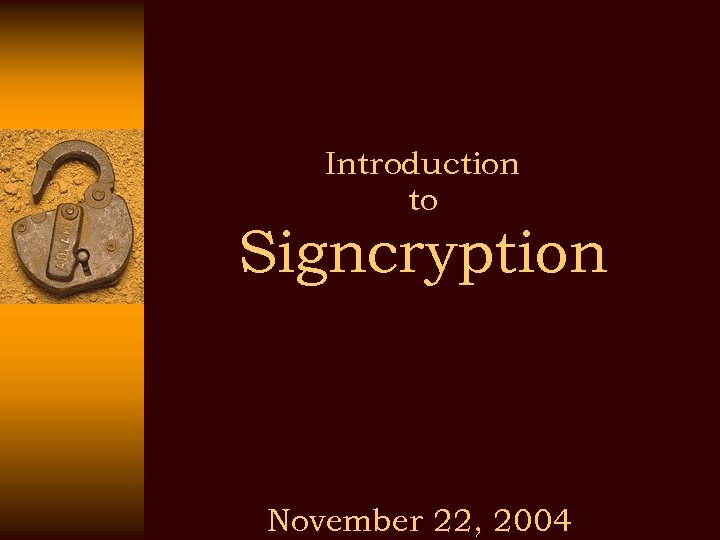Introduction to Signcryption November 22, 2004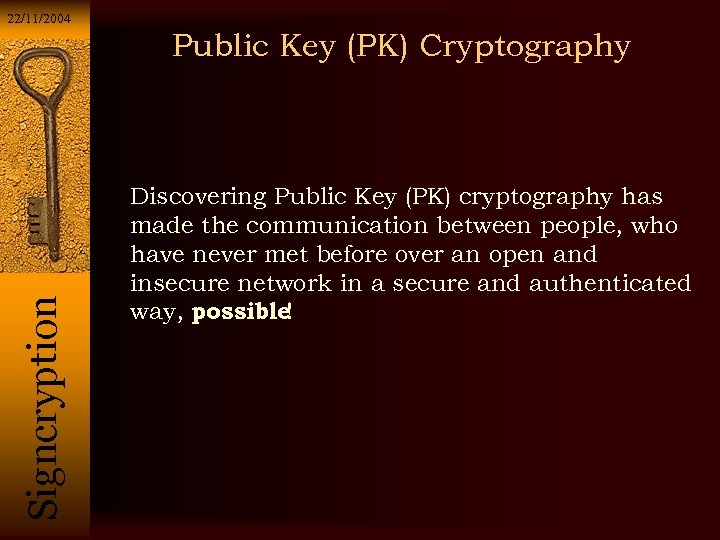22/11/2004 Si g n c r y p t i o n Public Key (PK) Cryptography Discovering Public Key (PK) cryptography has made the communication between people, who have never met before over an open and insecure network in a secure and authenticated way, possible !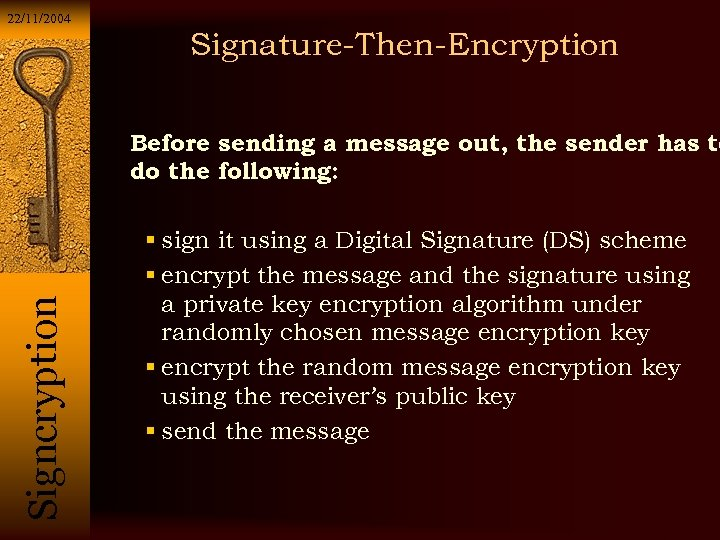22/11/2004 Signature-Then-Encryption Si g n c r y p t i o n Before sending a message out, the sender has to do the following: sign it using a Digital Signature (DS) scheme encrypt the message and the signature using a private key encryption algorithm under randomly chosen message encryption key encrypt the random message encryption key using the receiver’s public key send the message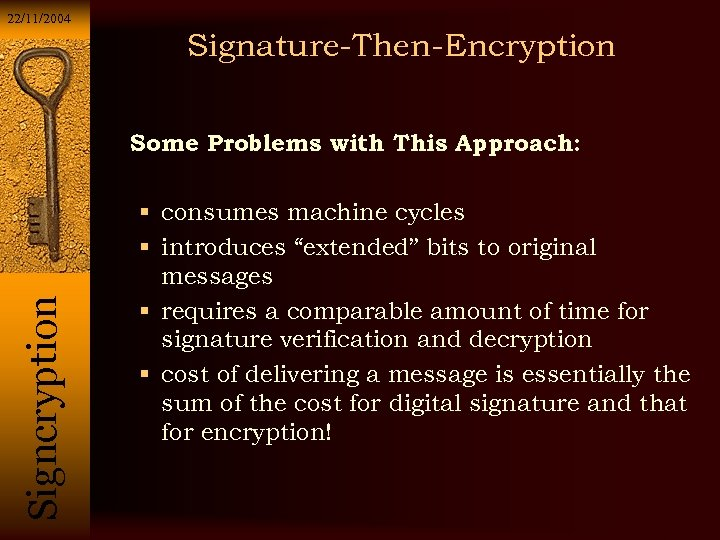22/11/2004 Signature-Then-Encryption Si g n c r y p t i o n Some Problems with This Approach: consumes machine cycles introduces “extended” bits to original messages requires a comparable amount of time for signature verification and decryption cost of delivering a message is essentially the sum of the cost for digital signature and that for encryption!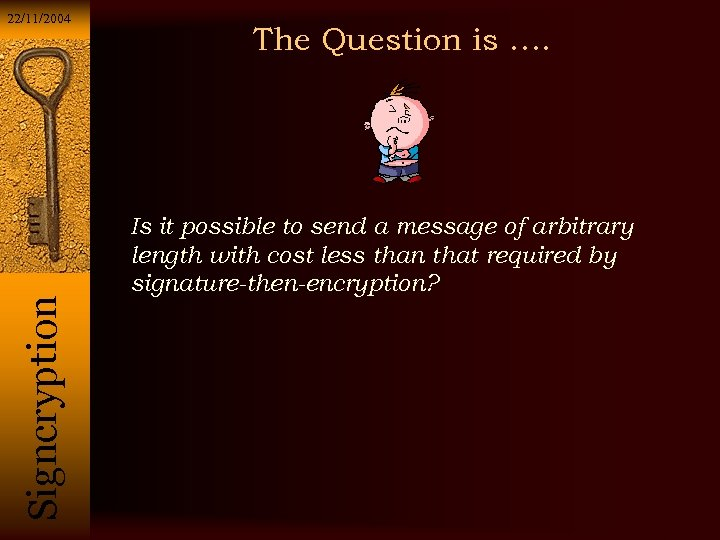Si g n c r y p t i o n 22/11/2004 The Question is …. Is it possible to send a message of arbitrary length with cost less than that required by signature-then-encryption?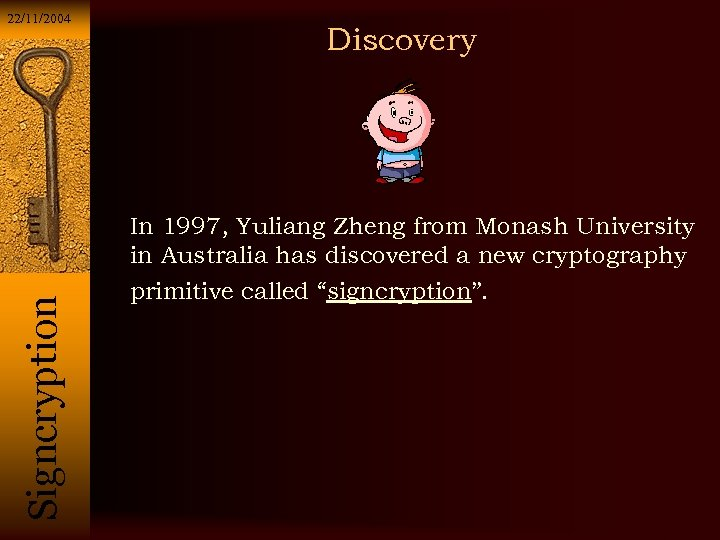22/11/2004 Discovery Si g n c r y p t i o n In 1997, Yuliang Zheng from Monash University in Australia has discovered a new cryptography primitive called “signcryption”.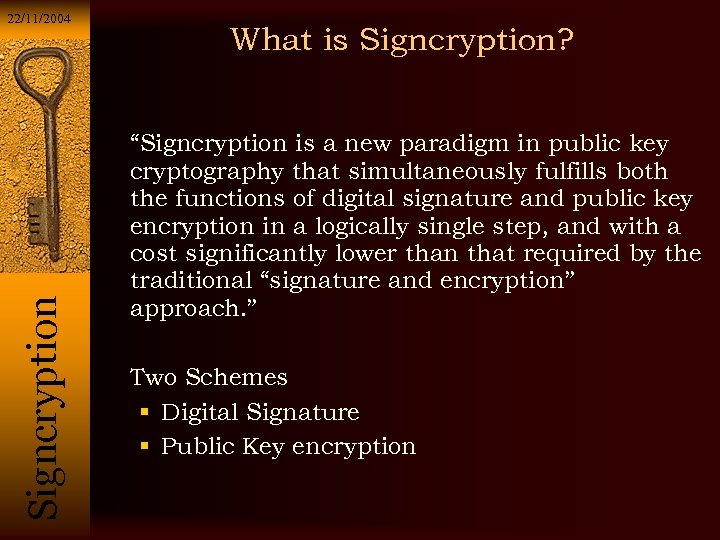Si g n c r y p t i o n 22/11/2004 What is Signcryption? “Signcryption is a new paradigm in public key cryptography that simultaneously fulfills both the functions of digital signature and public key encryption in a logically single step, and with a cost significantly lower than that required by the traditional “signature and encryption” approach. ” Two Schemes Digital Signature Public Key encryption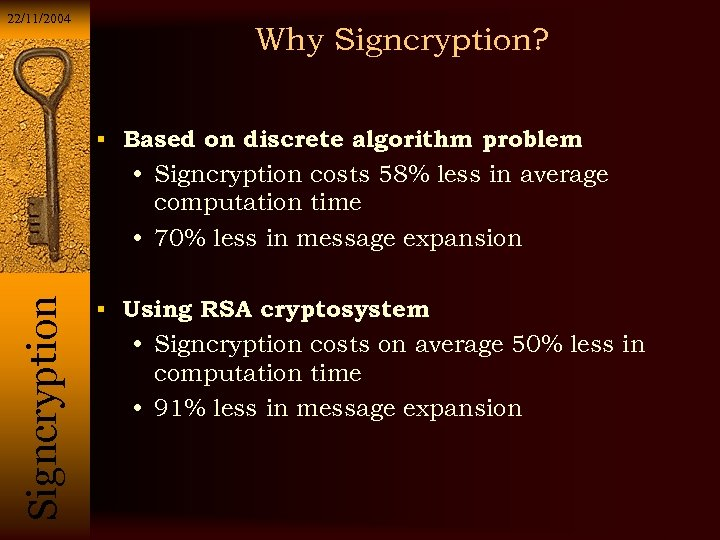22/11/2004 Why Signcryption? Based on discrete algorithm problem Si g n c r y p t i o n • Signcryption costs 58% less in average computation time • 70% less in message expansion Using RSA cryptosystem • Signcryption costs on average 50% less in computation time • 91% less in message expansion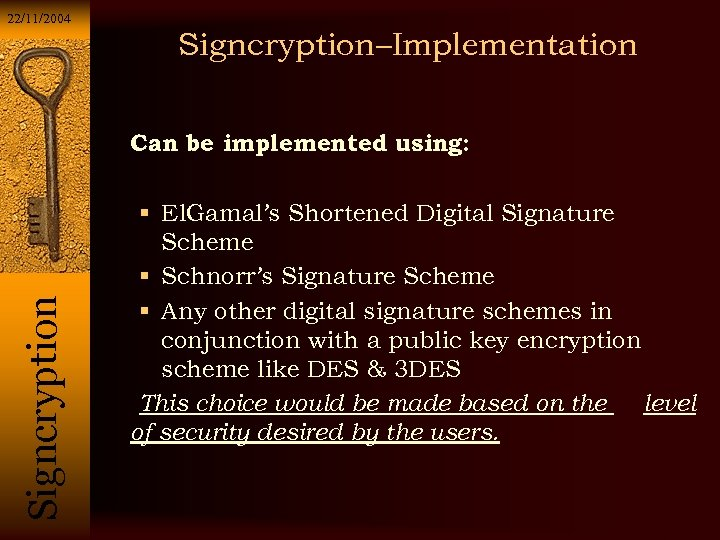22/11/2004 Signcryption–Implementation Si g n c r y p t i o n Can be implemented using: El. Gamal’s Shortened Digital Signature Scheme Schnorr’s Signature Scheme Any other digital signature schemes in conjunction with a public key encryption scheme like DES & 3 DES This choice would be made based on the level of security desired by the users.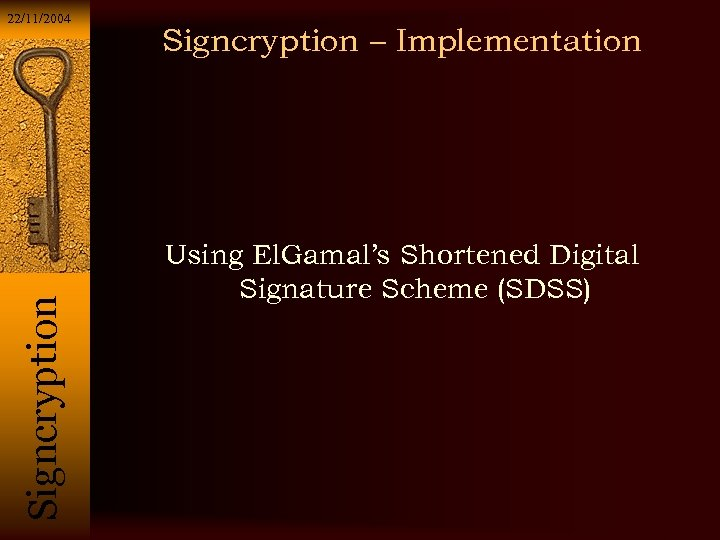Si g n c r y p t i o n 22/11/2004 Signcryption – Implementation Using El. Gamal’s Shortened Digital Signature Scheme (SDSS)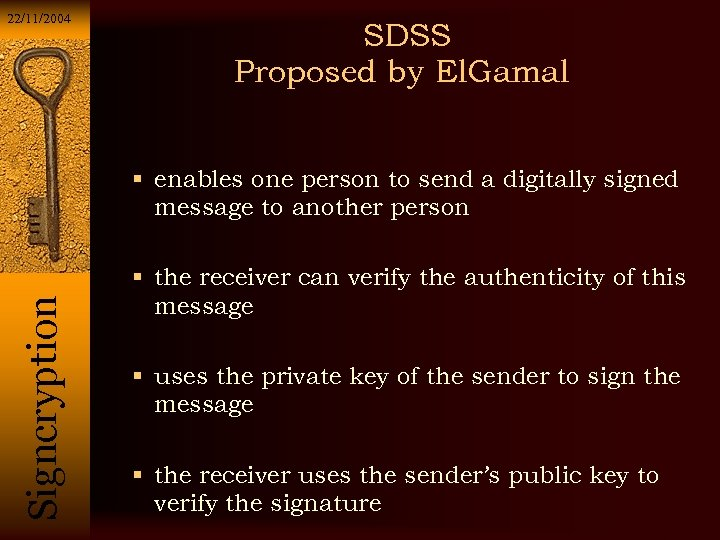22/11/2004 SDSS Proposed by El. Gamal Si g n c r y p t i o n enables one person to send a digitally signed message to another person the receiver can verify the authenticity of this message uses the private key of the sender to sign the message the receiver uses the sender’s public key to verify the signature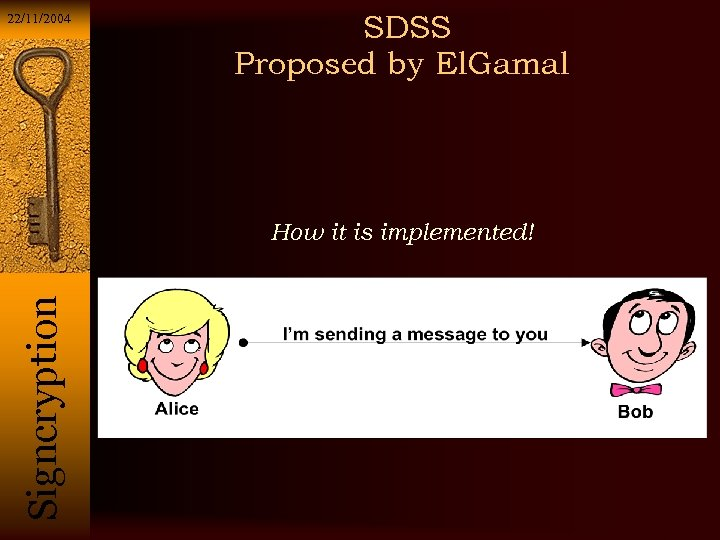22/11/2004 SDSS Proposed by El. Gamal Si g n c r y p t i o n How it is implemented!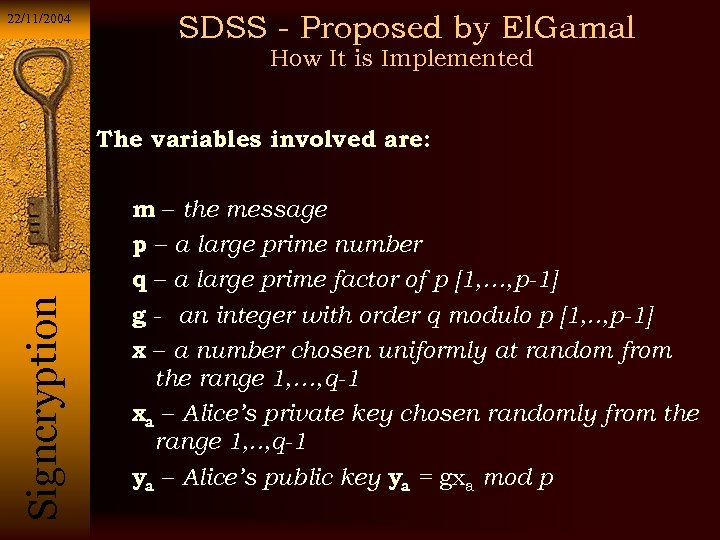22/11/2004 SDSS - Proposed by El. Gamal How It is Implemented Si g n c r y p t i o n The variables involved are: m – the message p – a large prime number q – a large prime factor of p [1, …, p-1] g - an integer with order q modulo p [1, . . , p-1] x – a number chosen uniformly at random from the range 1, …, q-1 xa – Alice’s private key chosen randomly from the range 1, . . , q-1 ya – Alice’s public key ya = gxa mod p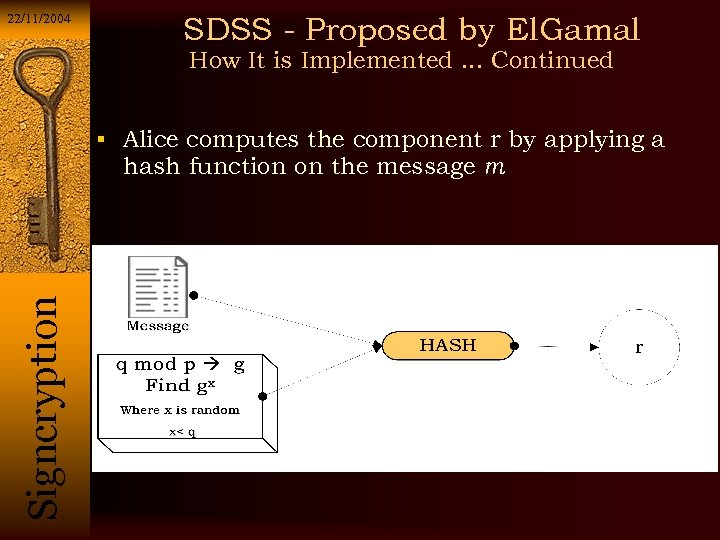22/11/2004 SDSS - Proposed by El. Gamal How It is Implemented. . . Continued Alice computes the component r by applying a Si g n c r y p t i o n hash function on the message m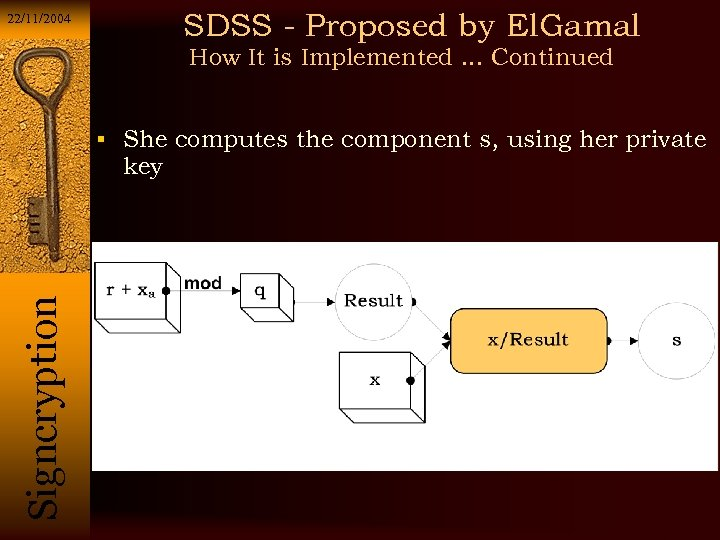SDSS - Proposed by El. Gamal 22/11/2004 How It is Implemented. . . Continued She computes the component s, using her private Si g n c r y p t i o n key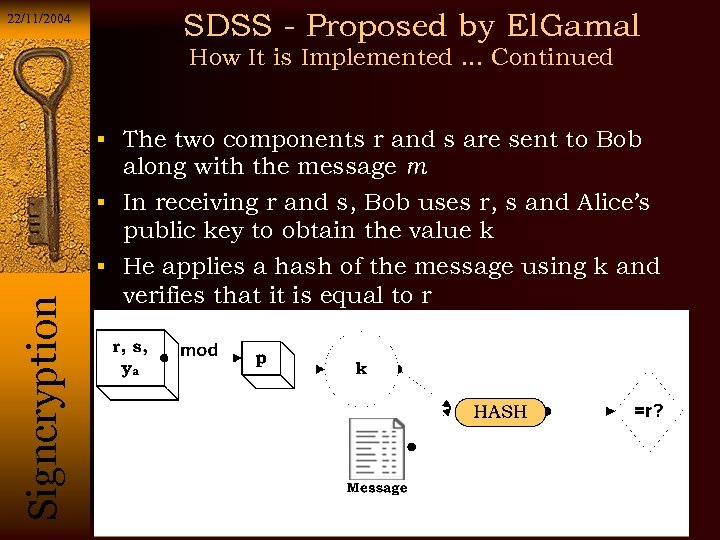22/11/2004 SDSS - Proposed by El. Gamal How It is Implemented. . . Continued The two components r and s are sent to Bob along with the message m In receiving r and s, Bob uses r, s and Alice’s public key to obtain the value k Si g n c r y p t i o n He applies a hash of the message using k and verifies that it is equal to r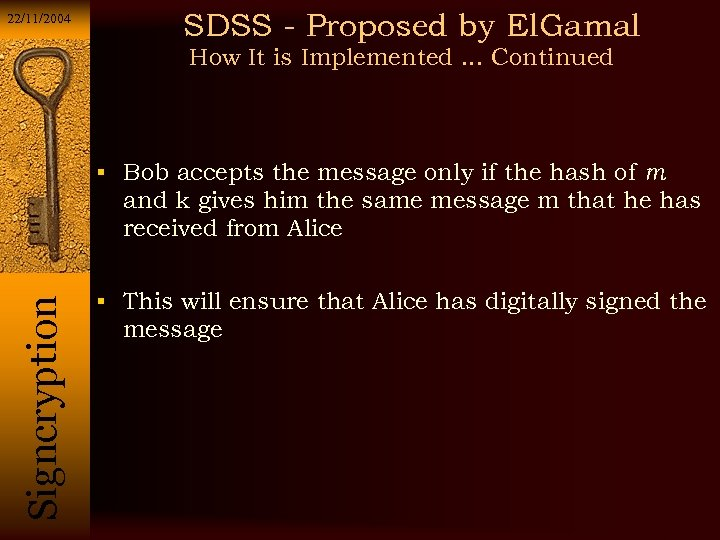22/11/2004 SDSS - Proposed by El. Gamal How It is Implemented. . . Continued Bob accepts the message only if the hash of m Si g n c r y p t i o n and k gives him the same message m that he has received from Alice This will ensure that Alice has digitally signed the message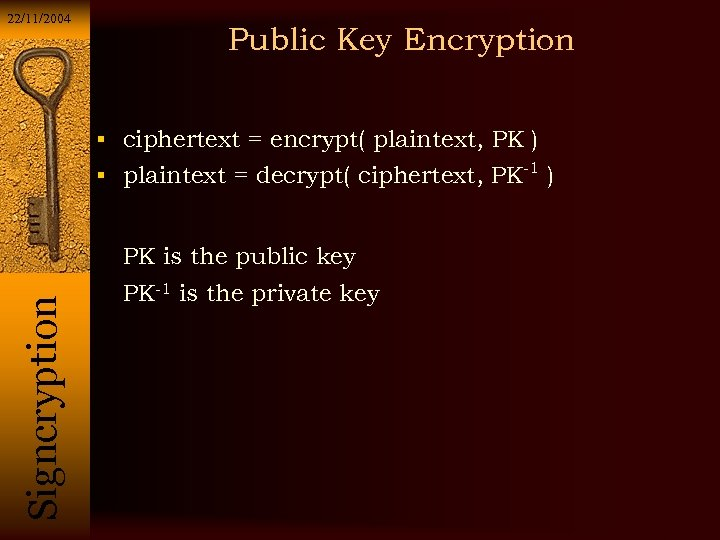22/11/2004 Public Key Encryption ciphertext = encrypt( plaintext, PK ) plaintext = decrypt( ciphertext, PK-1 ) Si g n c r y p t i o n PK is the public key PK-1 is the private key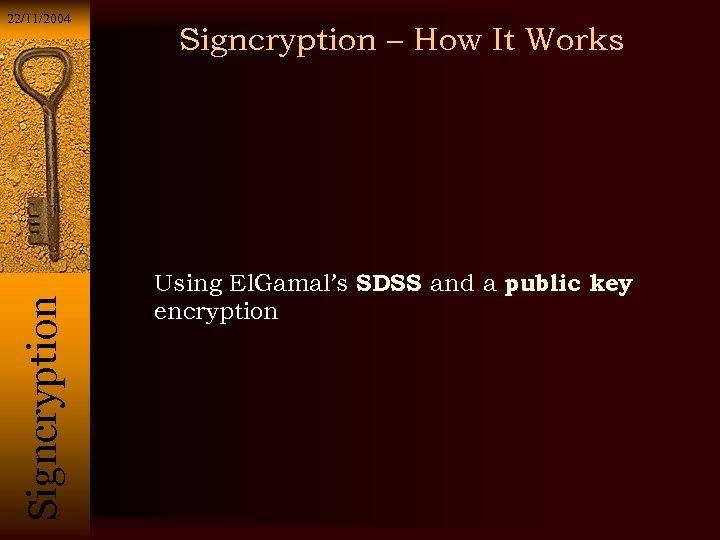Si g n c r y p t i o n 22/11/2004 Signcryption – How It Works Using El. Gamal’s SDSS and a public key encryption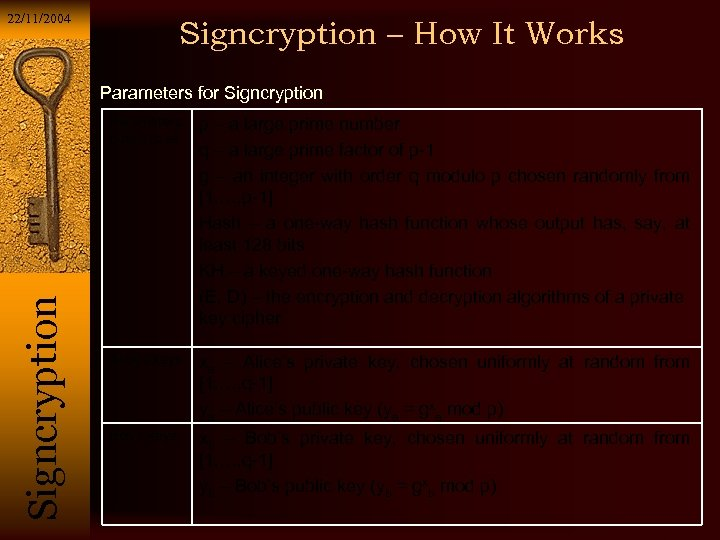22/11/2004 Signcryption – How It Works Parameters for Signcryption Si g n c r y p t i o n Parameters public to all p – a large prime number q – a large prime factor of p-1 g – an integer with order q modulo p chosen randomly from [1, …, p-1] Hash – a one-way hash function whose output has, say, at least 128 bits KH – a keyed one-way hash function (E, D) – the encryption and decryption algorithms of a private key cipher Alice’s keys xa – Alice’s private key, chosen uniformly at random from [1, …, q-1] ya – Alice’s public key (ya = gxa mod p) Bob’s keys xb – Bob’s private key, chosen uniformly at random from [1, …, q-1] yb – Bob’s public key (yb = gxb mod p)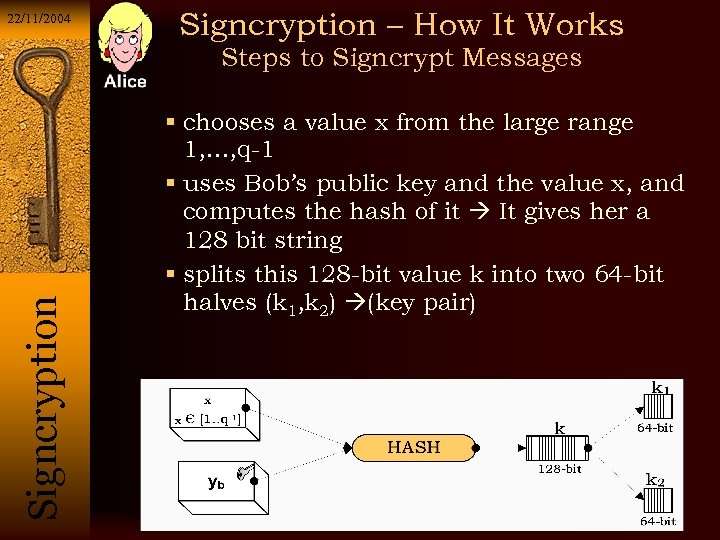22/11/2004 Signcryption – How It Works Si g n c r y p t i o n Steps to Signcrypt Messages chooses a value x from the large range 1, …, q-1 uses Bob’s public key and the value x, and computes the hash of it It gives her a 128 bit string splits this 128 -bit value k into two 64 -bit halves (k 1, k 2) (key pair)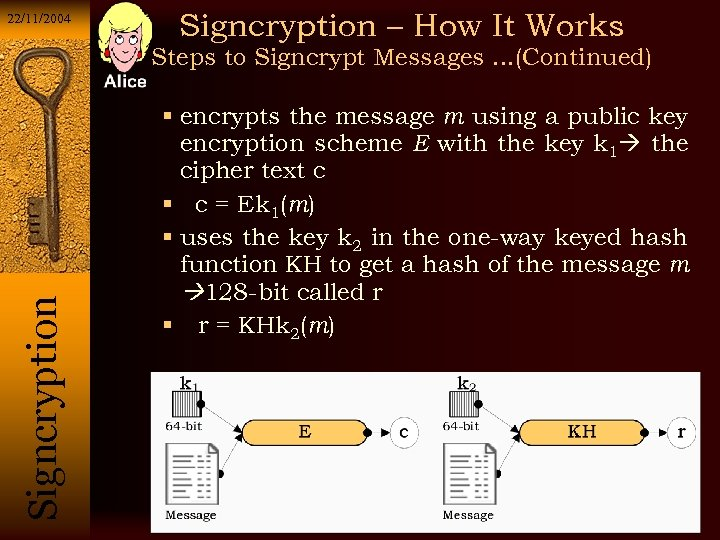22/11/2004 Signcryption – How It Works Si g n c r y p t i o n Steps to Signcrypt Messages. . . (Continued) encrypts the message m using a public key encryption scheme E with the key k 1 the cipher text c c = Ek 1(m) uses the key k 2 in the one-way keyed hash function KH to get a hash of the message m 128 -bit called r r = KHk 2(m)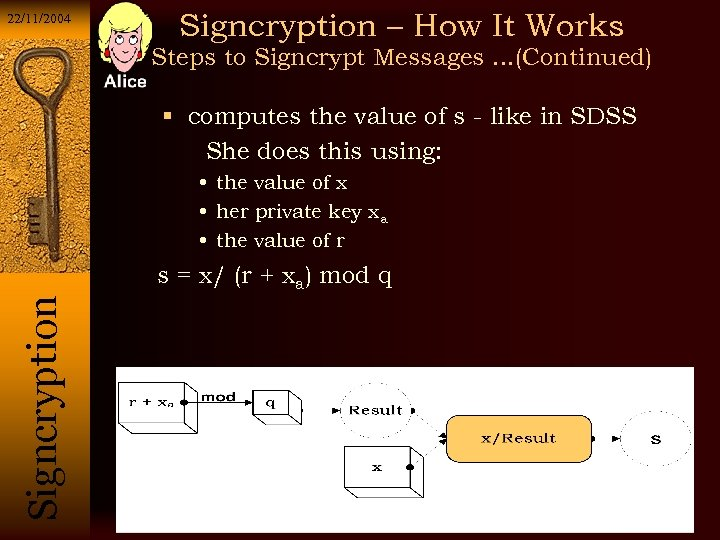22/11/2004 Signcryption – How It Works Steps to Signcrypt Messages. . . (Continued) computes the value of s - like in SDSS She does this using: • the value of x • her private key xa • the value of r Si g n c r y p t i o n s = x/ (r + xa) mod q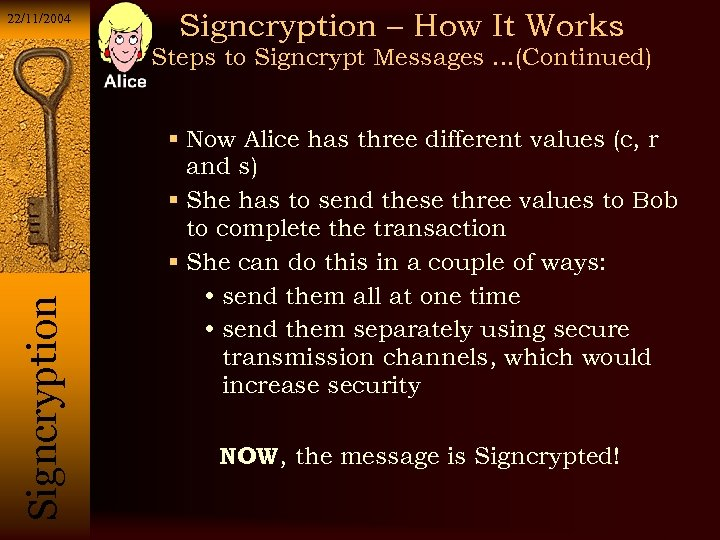22/11/2004 Signcryption – How It Works Si g n c r y p t i o n Steps to Signcrypt Messages. . . (Continued) Now Alice has three different values (c, r and s) She has to send these three values to Bob to complete the transaction She can do this in a couple of ways: • send them all at one time • send them separately using secure transmission channels, which would increase security NOW, the message is Signcrypted!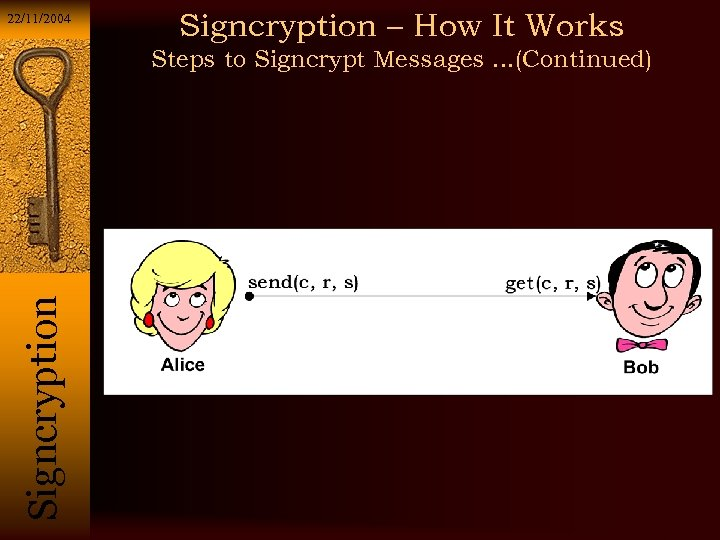22/11/2004 Signcryption – How It Works Si g n c r y p t i o n Steps to Signcrypt Messages. . . (Continued)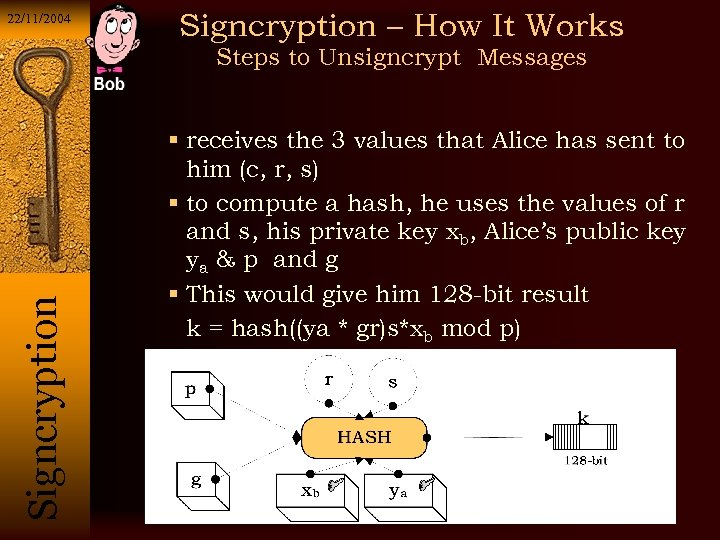22/11/2004 Signcryption – How It Works Si g n c r y p t i o n Steps to Unsigncrypt Messages receives the 3 values that Alice has sent to him (c, r, s) to compute a hash, he uses the values of r and s, his private key xb, Alice’s public key ya & p and g This would give him 128 -bit result k = hash((ya * gr)s*xb mod p)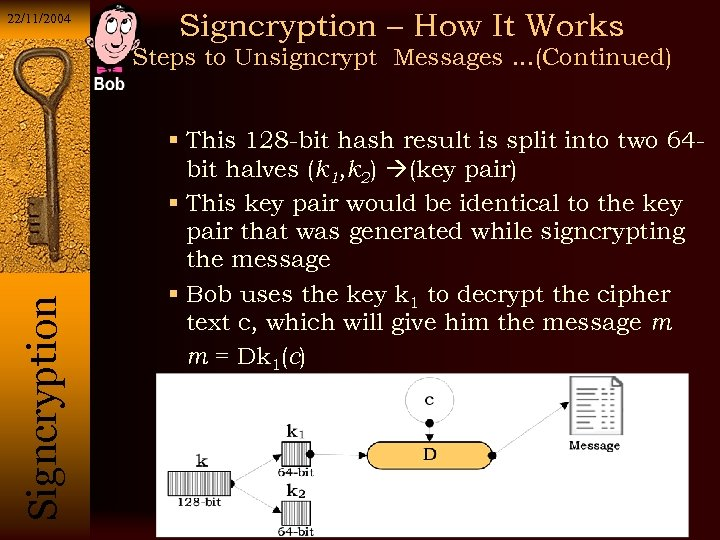22/11/2004 Signcryption – How It Works Si g n c r y p t i o n Steps to Unsigncrypt Messages. . . (Continued) This 128 -bit hash result is split into two 64 bit halves (k 1, k 2) (key pair) This key pair would be identical to the key pair that was generated while signcrypting the message Bob uses the key k 1 to decrypt the cipher text c, which will give him the message m m = Dk 1(c)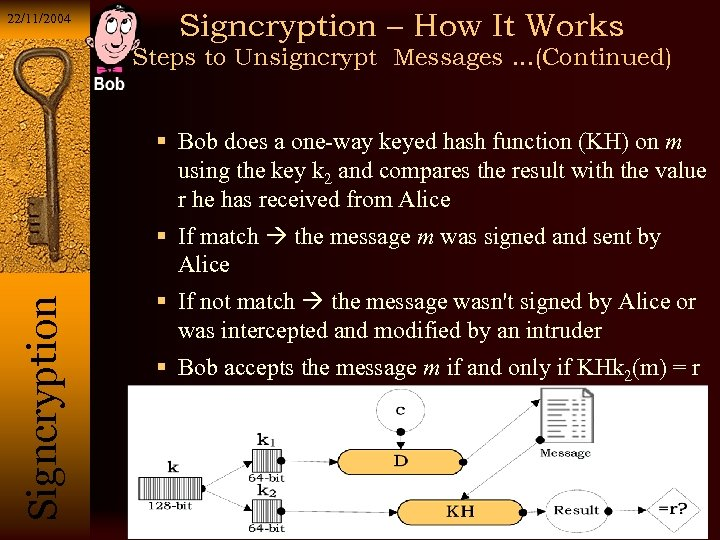22/11/2004 Signcryption – How It Works Steps to Unsigncrypt Messages. . . (Continued) Bob does a one-way keyed hash function (KH) on m using the key k 2 and compares the result with the value r he has received from Alice If match the message m was signed and sent by Si g n c r y p t i o n Alice If not match the message wasn't signed by Alice or was intercepted and modified by an intruder Bob accepts the message m if and only if KHk 2(m) = r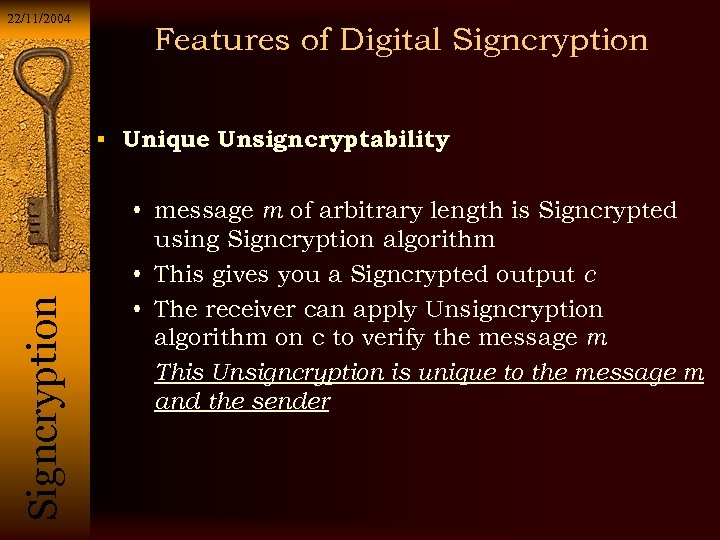22/11/2004 Features of Digital Signcryption Unique Unsigncryptability Si g n c r y p t i o n • message m of arbitrary length is Signcrypted using Signcryption algorithm • This gives you a Signcrypted output c • The receiver can apply Unsigncryption algorithm on c to verify the message m This Unsigncryption is unique to the message m and the sender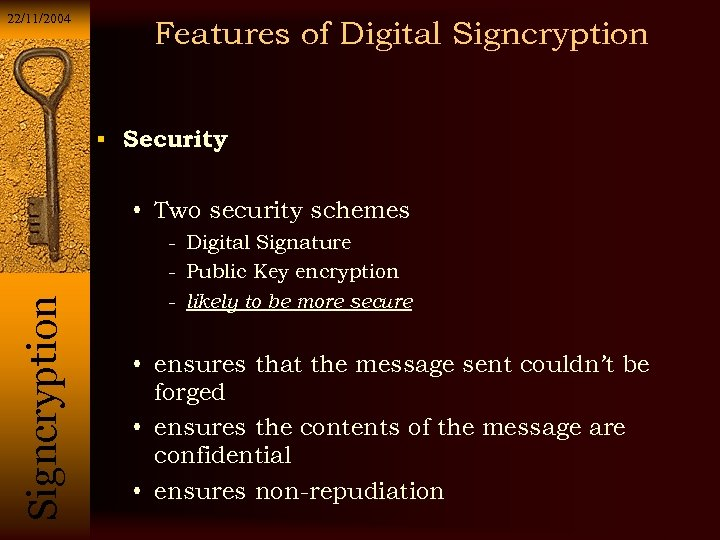22/11/2004 Features of Digital Signcryption Security • Two security schemes - Digital Signature Si g n c r y p t i o n - Public Key encryption - likely to be more secure • ensures that the message sent couldn’t be forged • ensures the contents of the message are confidential • ensures non-repudiation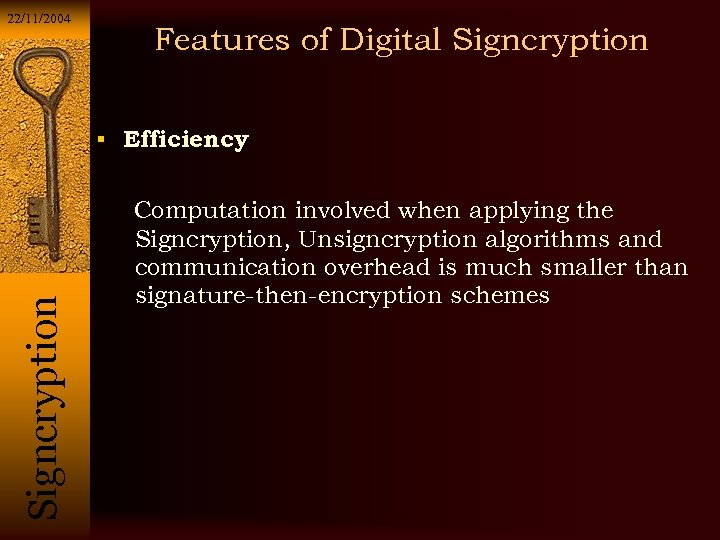22/11/2004 Features of Digital Signcryption Si g n c r y p t i o n Efficiency Computation involved when applying the Signcryption, Unsigncryption algorithms and communication overhead is much smaller than signature-then-encryption schemes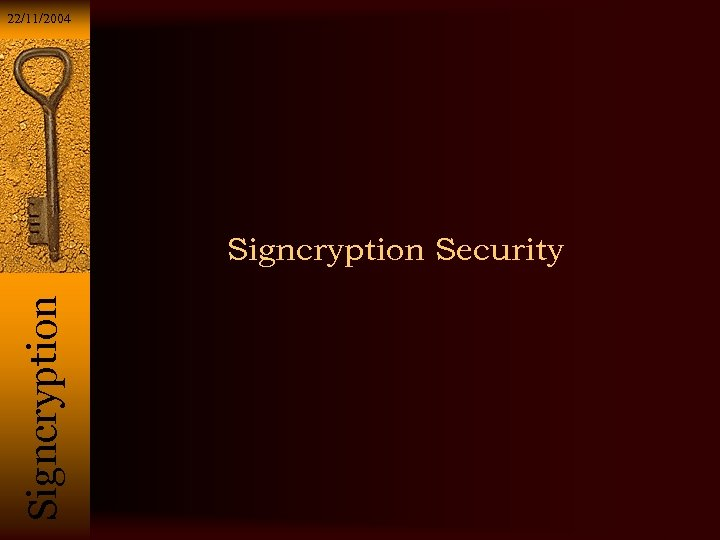22/11/2004 Si g n c r y p t i o n Signcryption Security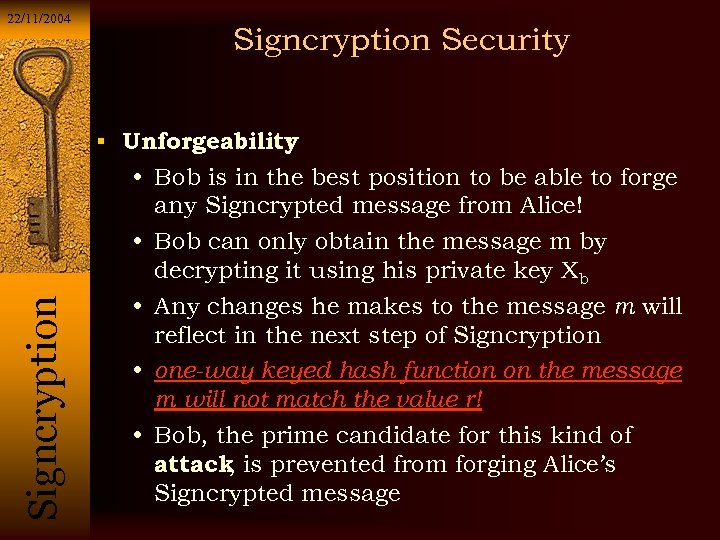22/11/2004 Signcryption Security Si g n c r y p t i o n Unforgeability : • Bob is in the best position to be able to forge any Signcrypted message from Alice! • Bob can only obtain the message m by decrypting it using his private key Xb • Any changes he makes to the message m will reflect in the next step of Signcryption • one-way keyed hash function on the message m will not match the value r! • Bob, the prime candidate for this kind of attack is prevented from forging Alice’s , Signcrypted message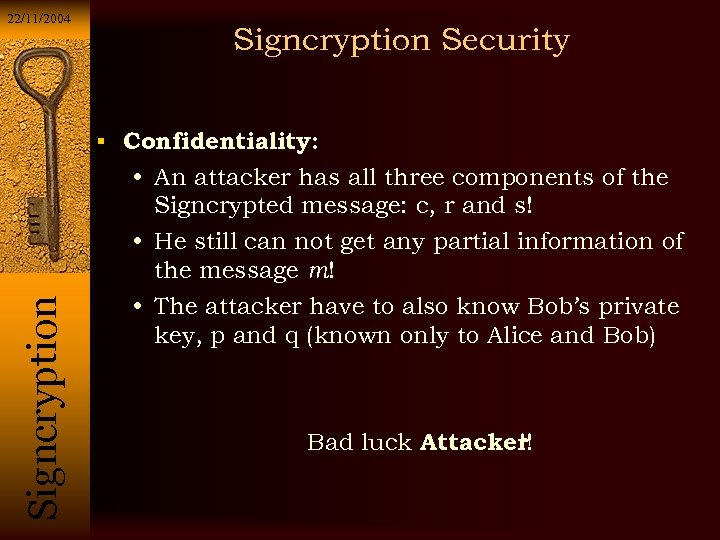22/11/2004 Signcryption Security Si g n c r y p t i o n Confidentiality: • An attacker has all three components of the Signcrypted message: c, r and s! • He still can not get any partial information of the message m! • The attacker have to also know Bob’s private key, p and q (known only to Alice and Bob) Bad luck Attacker! !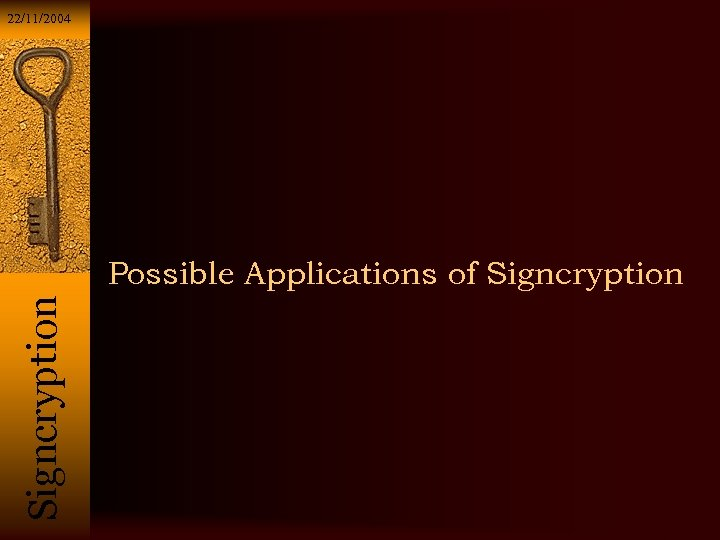22/11/2004 Si g n c r y p t i o n Possible Applications of Signcryption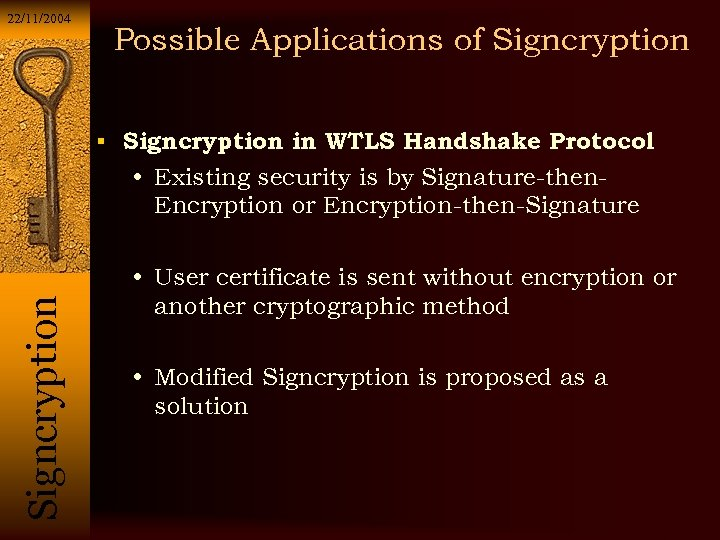22/11/2004 Possible Applications of Signcryption in WTLS Handshake Protocol Si g n c r y p t i o n • Existing security is by Signature-then. Encryption or Encryption-then-Signature • User certificate is sent without encryption or another cryptographic method • Modified Signcryption is proposed as a solution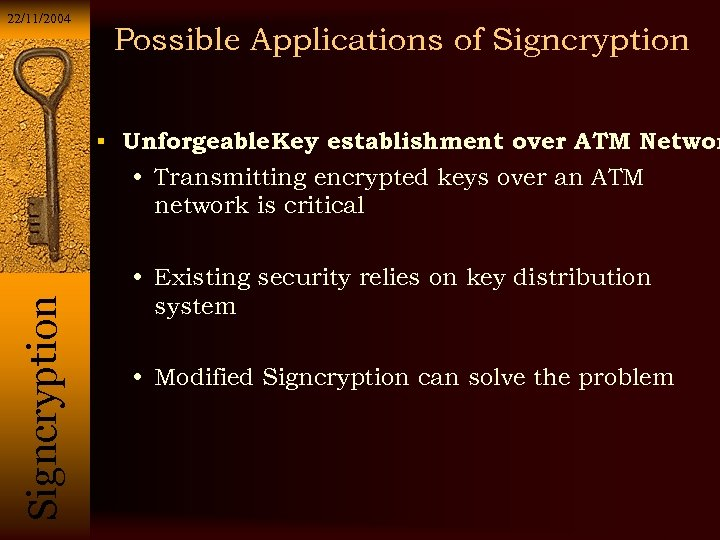22/11/2004 Possible Applications of Signcryption Unforgeable Key establishment over ATM Networ Si g n c r y p t i o n • Transmitting encrypted keys over an ATM network is critical • Existing security relies on key distribution system • Modified Signcryption can solve the problem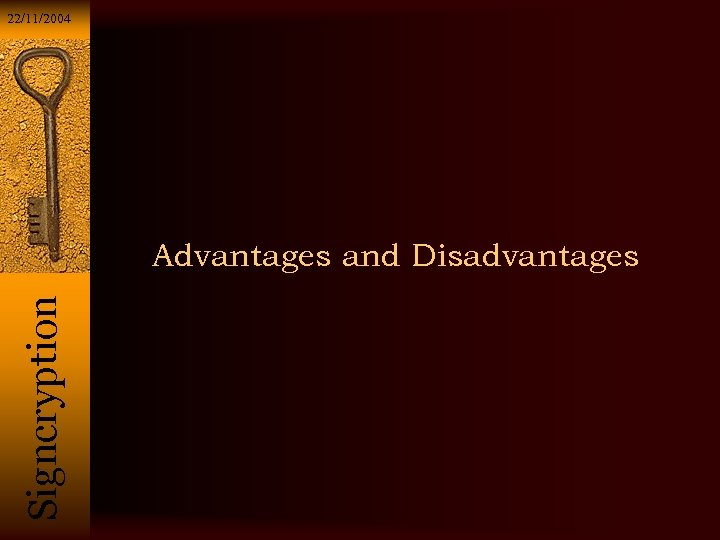22/11/2004 Si g n c r y p t i o n Advantages and Disadvantages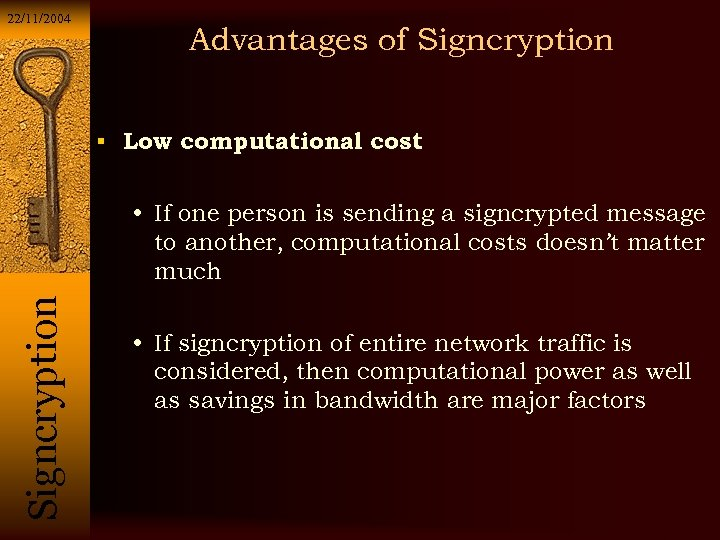22/11/2004 Advantages of Signcryption Low computational cost Si g n c r y p t i o n • If one person is sending a signcrypted message to another, computational costs doesn’t matter much • If signcryption of entire network traffic is considered, then computational power as well as savings in bandwidth are major factors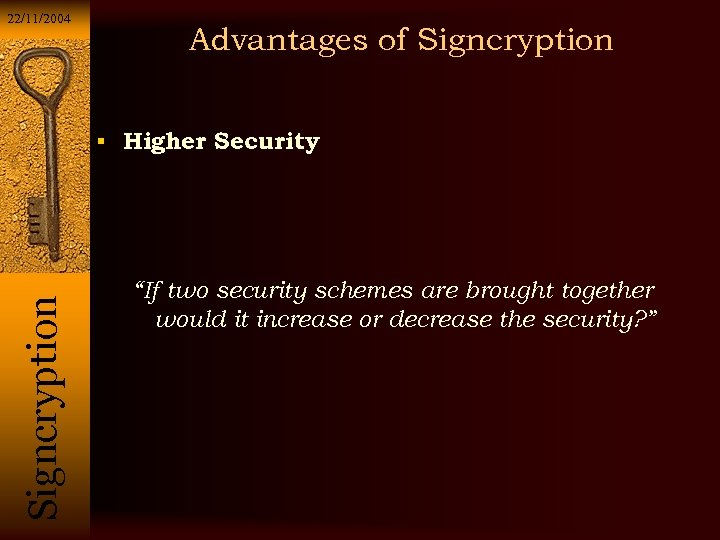22/11/2004 Advantages of Signcryption Si g n c r y p t i o n Higher Security “If two security schemes are brought together would it increase or decrease the security? ”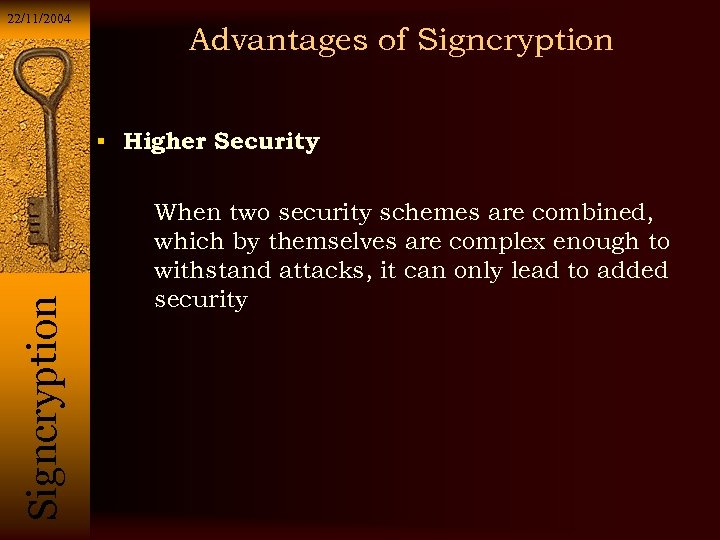22/11/2004 Advantages of Signcryption Si g n c r y p t i o n Higher Security When two security schemes are combined, which by themselves are complex enough to withstand attacks, it can only lead to added security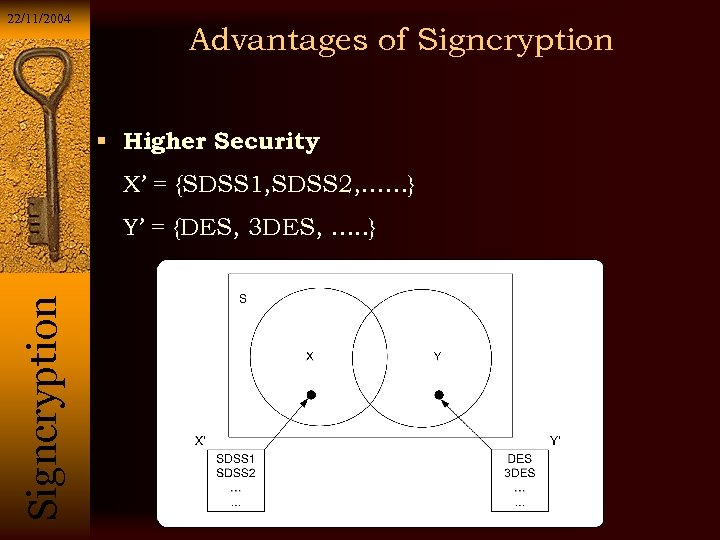22/11/2004 Advantages of Signcryption Higher Security X’ = {SDSS 1, SDSS 2, ……} Si g n c r y p t i o n Y’ = {DES, 3 DES, …. . }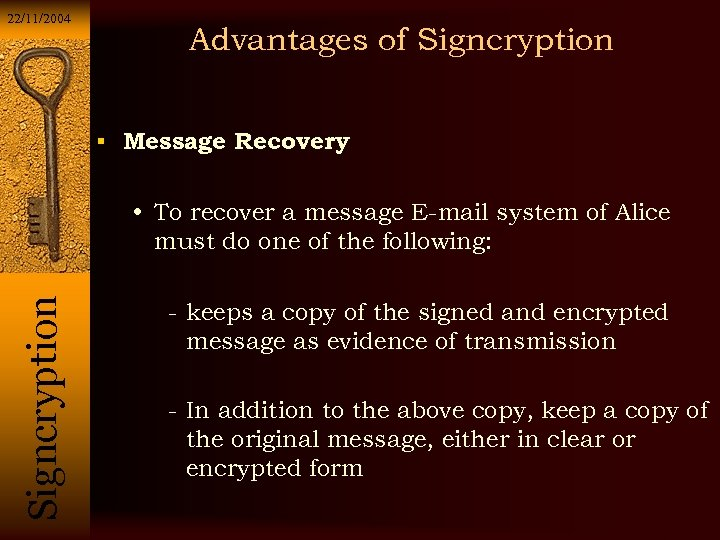22/11/2004 Advantages of Signcryption Message Recovery Si g n c r y p t i o n • To recover a message E-mail system of Alice must do one of the following: - keeps a copy of the signed and encrypted message as evidence of transmission - In addition to the above copy, keep a copy of the original message, either in clear or encrypted form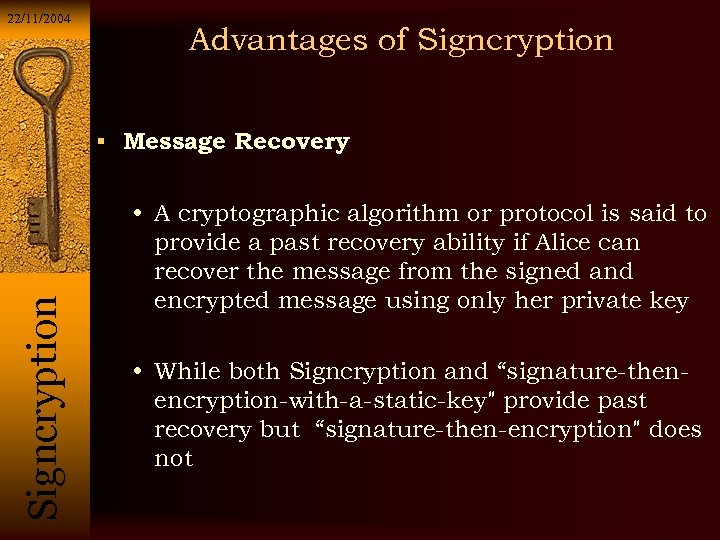22/11/2004 Advantages of Signcryption Si g n c r y p t i o n Message Recovery • A cryptographic algorithm or protocol is said to provide a past recovery ability if Alice can recover the message from the signed and encrypted message using only her private key • While both Signcryption and “signature-thenencryption-with-a-static-key" provide past recovery but “signature-then-encryption" does not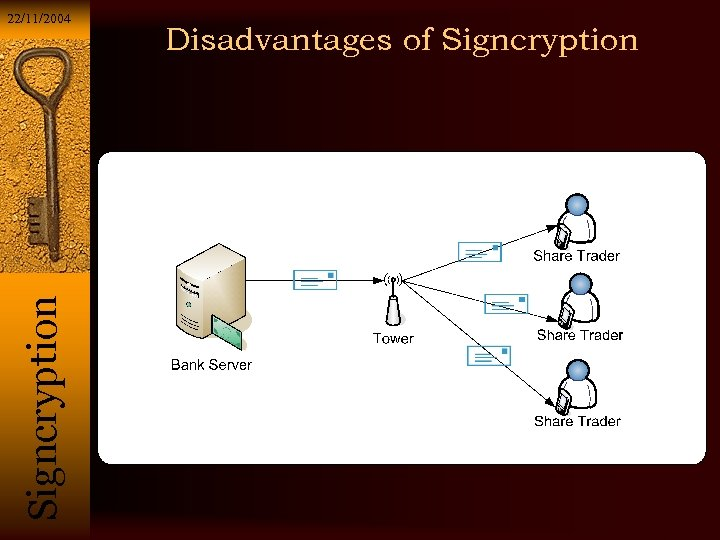Si g n c r y p t i o n 22/11/2004 Disadvantages of Signcryption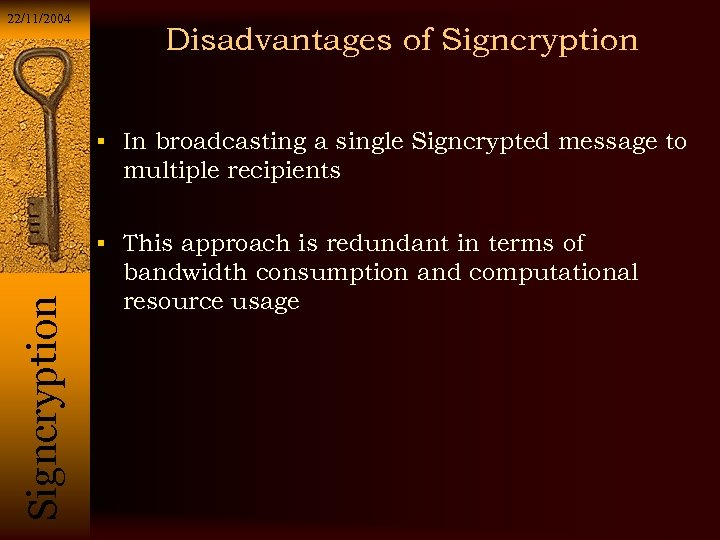22/11/2004 Disadvantages of Signcryption In broadcasting a single Signcrypted message to multiple recipients Si g n c r y p t i o n This approach is redundant in terms of bandwidth consumption and computational resource usage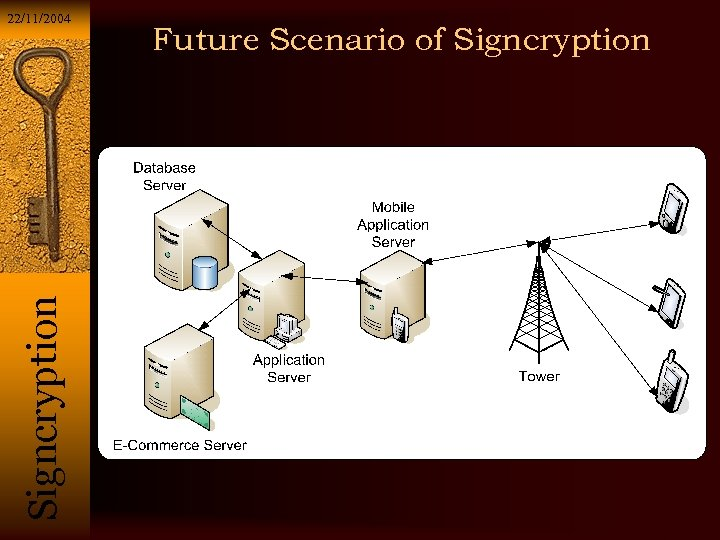Si g n c r y p t i o n 22/11/2004 Future Scenario of Signcryption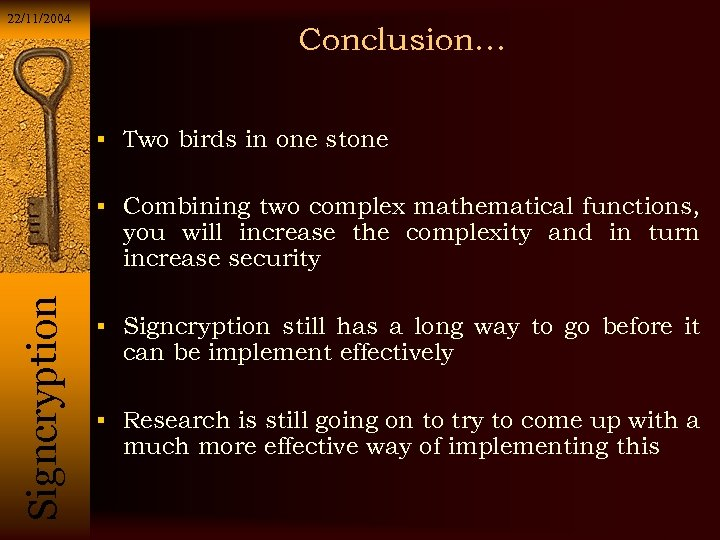22/11/2004 Conclusion… Two birds in one stone Combining two complex mathematical functions, Si g n c r y p t i o n you will increase the complexity and in turn increase security Signcryption still has a long way to go before it can be implement effectively Research is still going on to try to come up with a much more effective way of implementing this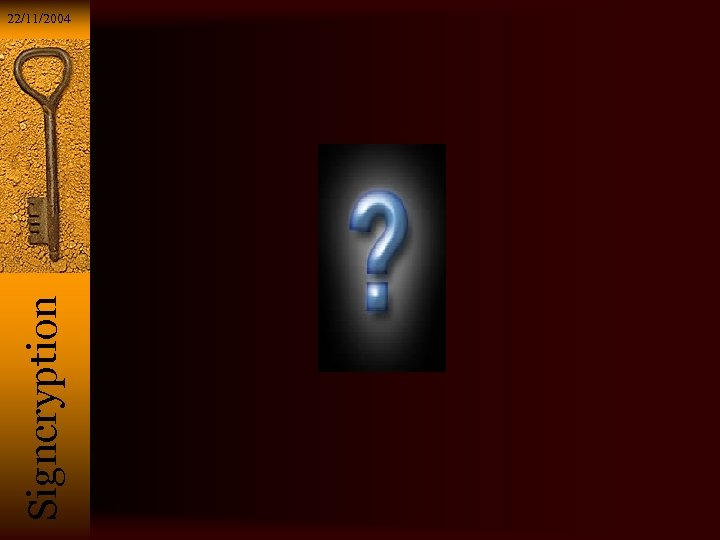Si g n c r y p t i o n 22/11/2004